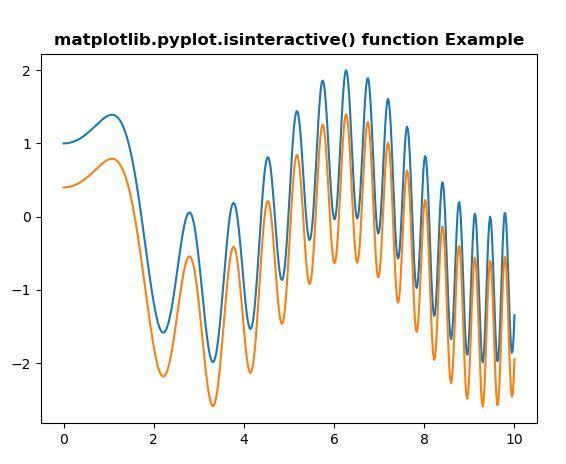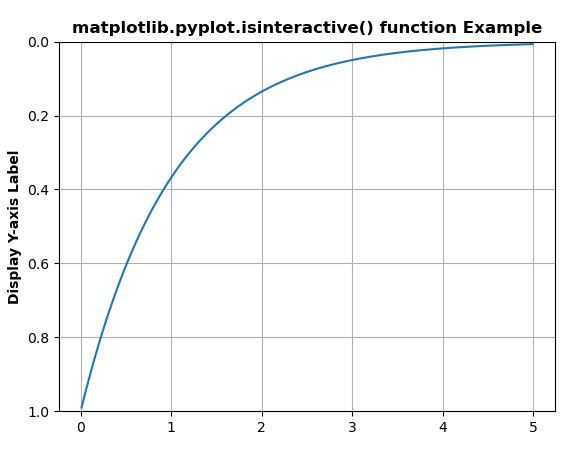# Matplotlib.pyplot.isinteractive() in Python

• Last Updated : 10 May, 2020

Matplotlib is a library in Python and it is numerical – mathematical extension for NumPy library. Pyplot is a state-based interface to a Matplotlib module which provides a MATLAB-like interface. There are various plots which can be used in Pyplot are Line Plot, Contour, Histogram, Scatter, 3D Plot, etc.

## matplotlib.pyplot.isinteractive() method

Attention geek! Strengthen your foundations with the Python Programming Foundation Course and learn the basics.

To begin with, your interview preparations Enhance your Data Structures concepts with the Python DS Course. And to begin with your Machine Learning Journey, join the Machine Learning - Basic Level Course

The isinteractive() method in pyplot module of matplotlib library is used to get whether to redraw after every plotting command.

Syntax: matplotlib.pyplot.isinteractive()

Parameters: This method does not accept any parameters.

Returns: This method returns whether to redraw after every plotting command or not.

Below examples illustrate the matplotlib.pyplot.isinteractive() function in matplotlib.pyplot:

Example 1:

 `# Implementation of matplotlib function``import` `numpy as np``import` `matplotlib.pyplot as plt``     ` ` ` `x ``=` `np.linspace(``0``, ``10``, ``500``)``y ``=` `np.sin(x``*``*``2``)``+``np.cos(x)``     ` `plt.plot(x, y, label ``=``'Line 1'``)``     ` `plt.plot(x, y ``-` `0.6``, label ``=``'Line 2'``)``   ` `w ``=` `plt.isinteractive()``   ` `print``(``"Is we can redraw after every plotting command : "``,``       ``str``(w))``      ` `plt.title('matplotlib.pyplot.isinteractive() function \``Example', fontweight ``=``"bold"``) `` ` `plt.show()`

Output:```Is we can redraw after every plotting command :  False
```

Example 2:

 `# Implementation of matplotlib function``import` `matplotlib.pyplot as plt``import` `numpy as np`` ` `      ` `t ``=` `np.arange(``0.01``, ``5.0``, ``0.01``)``s ``=` `np.exp(``-``t)``      ` `plt.plot(t, s)``plt.ylim(``1``, ``0``)``plt.ylabel(``'Display Y-axis Label'``, fontweight ``=``'bold'``)``plt.grid(``True``)``   ` `w ``=` `plt.isinteractive()``   ` `print``(``"Is we can redraw after every plotting command : "``,``       ``str``(w))``      ` `plt.title('matplotlib.pyplot.isinteractive() function \``Example', fontweight ``=``"bold"``) `` ` `plt.show()`

Output:```Is we can redraw after every plotting command :  False
```

My Personal Notes arrow_drop_up